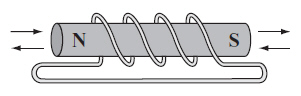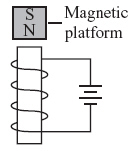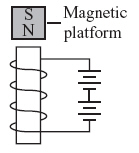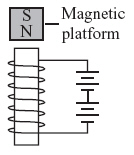Name:    MCAS Review Ch. 16-17 Magnetism, Electromagnets and Induction

Multiple Choice
Identify the choice that best completes the statement or answers the question.

1.

A magnetic compass is placed near an insulated copper wire. When the wire is connected to a battery, the compass needle changes position. Which of the following is the best explanation for the movement of the needle? (MCAS 2008)
 a. The copper wire magnetizes the needle to create a force. b. The needle magnetizes the copper wire to create a force. c. The current in the wire produces a magnetic field and exerts a force on the needle. d. The insulation on the wire becomes energized and exerts a force on the needle.

2.

The diagram below shows copper wire wrapped around a cardboard tube, which is then attached to a galvanometer. A galvanometer detects and measures small amounts of electric current.Which of the following would cause the galvanometer needle to move? (MCAS 2010)
 a. wrapping additional wire around the tube b. uncoiling the wire wrapped around the tube c. moving a magnet back and forth inside the tube d. moving an aluminum block up and down inside the tube

3.

The diagram below shows a bar magnet within a coil of conducting wire. The magnet is moved back and forth. The magnet never has contact with the wire. (MCAS 2012)As the magnet is moved, the wire will
 a. decrease in temperature. c. demagnetize the bar magnet. b. become negatively charged. d. have an electric current.

4.

Which of the following statements describes an electric generator? (MCAS 2013)
 a. A magnet is rotated through a coil of wire to produce an electric current. b. Electric potential in a rotating coil of wire creates a permanent magnet. c. An electrical current causes a coil of wire to rotate in a magnetic field. d. Forces from a permanent magnet allow a coil of wire to rotate.

5.

Precise measuring instruments require shock absorbers to eliminate small vibrations that can affect the results of an experiment. One type of shock absorber that can be used is an electromagnet that repels a magnetic platform placed above it. Which of the following setups would provide the greatest lift to the platform? (MCAS 2009)
 a.c.b.d.6.

Which of the following forces allow a battery-powered motor to generate mechanical energy? (MCAS 2014)
 a. magnetic and static c. static and gravitational b. electric and magnetic d. electric and gravitational

7.

What is the purpose of the wire coil in an electromagnet? (MCAS 2014)
 a. to hold the battery in its center b. to decrease the diameter of the wire c. to decrease the resistance of the wire d. to concentrate the magnetic field in its center